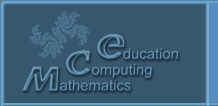Русский
 ! Вопросы и ответы

# Proceedings

## Kolmogorov foundations of mathematics and education

Kuzichev A. S.

Russia, Moscow

In foundations of mathematics are distinguished two way of constructing of first-order theories: the well-known way of Frege and the new way of Kolmogorov. Various postulates (axiomes and derivation rules) of all theories (calculi) are formulated on the way of Frege. The author realize the Kolmogorov set-theoretic reconstruction of basis concepts of calculi constructed on the way of Frege by Mendelson. Following Kolmogorov, the central concept of such theory is the infinite class of derivations, but not the finite derivation as it is usual beginning with Frege. On Kolmogorov set-theoretic way for the first time is obtained the proof of the consistency of all known (on Frege way) first-order theories, reducable into propositional logic. The proof is obtained for each such incomplete (according to Goedel) theory by known school combinatorial means. The results of this paper can be and must be introduce into educational process – to teach the foundations of sciences is advisable not by Frege with restrictive Goedel incompleteness theorems (as it is usual today), but by Kolmogorov set-theoretically without restrictions.

© 2004 Designed by Lyceum of Informational Technologies №1533